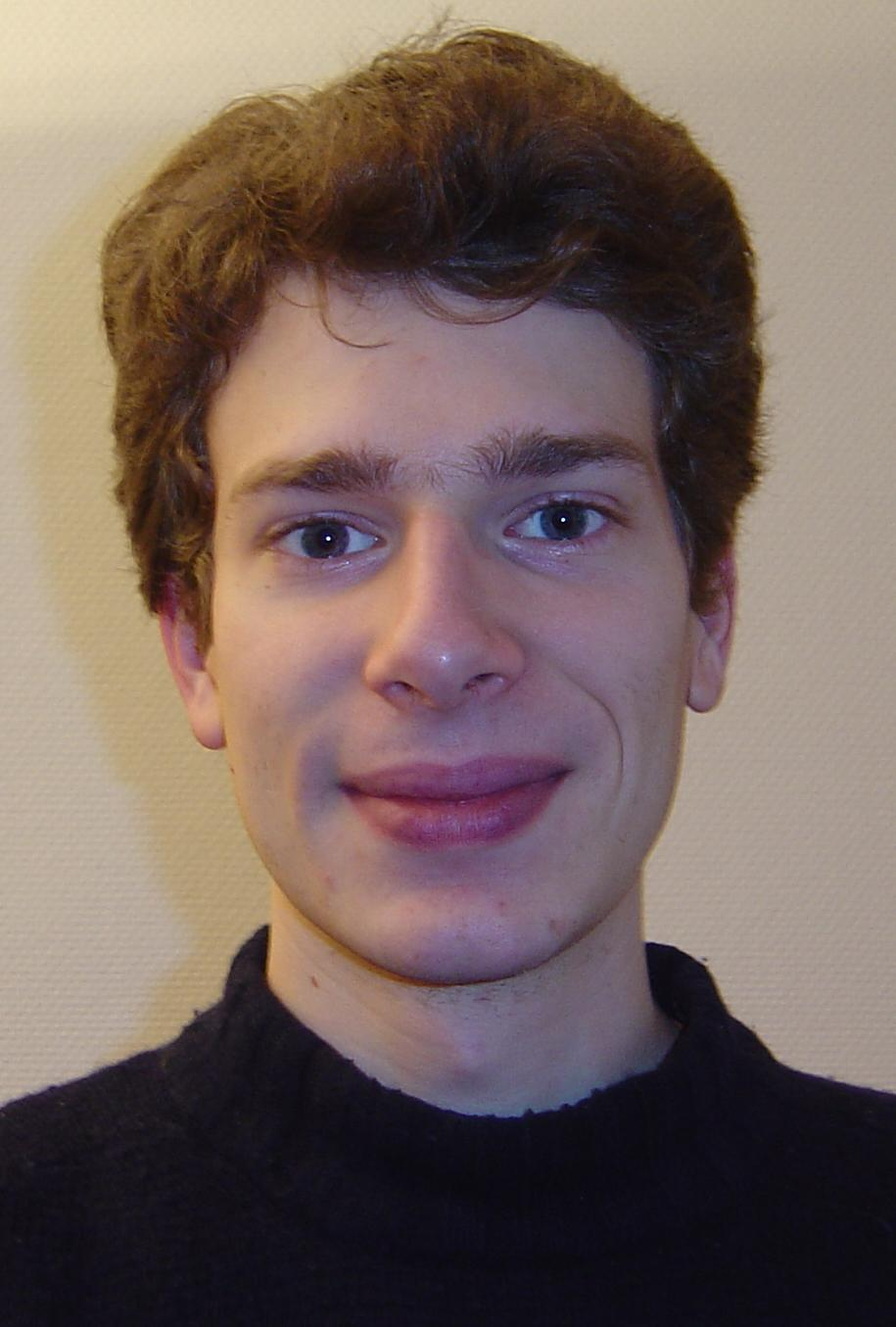Raphaël Côte

CNRS Research Fellow

Centre de Mathématiques Laurent Schwartz UMR 7640
École Polytechnique
91128 Palaiseau Cedex - France

 Email : Office : 10 06 Phone : +33 1 69 33 49 54 Fax : +33 1 69 33 49 49

Research

Research themes

• Non-linear evolution PDEs.
• Dynamics, stability, blow-up, asymptotic behaviour and classification of PDEs.
• Wave equations, generalized Korteweg-de Vries equations, non-linear Schrödinger equations, dispersive equations.
• Micromagnetism, Landau-Lifshitz-Gilbert equation, Néel walls.

Teaching

I am Professeur chargé de cours at the École polytechnique (teaching position).
• Problem sessions for the class MAT431: Differential equations and dynamical systems (Raphaël Krikorian).
• Problem sessions for the class MAT433: Distributions, Fourier and Partial Differential Equations (François Golse).

Funding

• ERC Blowup, Dispersion and Solitons (Advanced Grant 291214). Principal investigator: Frank Merle.
• ANR MAToS (Mathematical Analysis of Topological Singularities in some physical problems). Principal investigator: Radu Ignat.

`Last change: December 2014. `